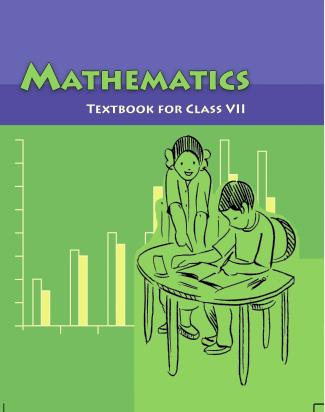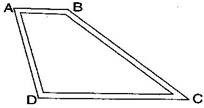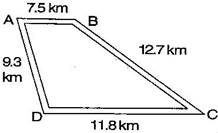1. /
2. CBSE
3. /
4. Class 07
5. /
6. Mathematics
7. /
8. NCERT Solutions for Class...

# NCERT Solutions for Class 7 Maths Exercise 2.5### myCBSEguide App

Download the app to get CBSE Sample Papers 2023-24, NCERT Solutions (Revised), Most Important Questions, Previous Year Question Bank, Mock Tests, and Detailed Notes.

NCERT solutions for Maths Fractions and Decimals## NCERT Solutions for Class 7 Maths Fractions and Decimals

###### Question 1.Which is greater:

(i) 0.5 or 0.05

(ii) 0.7 or 0.5

(iii) 7 or 0.7

(iv) 1.37 or 1.49

(v) 2.03 or 2.30

(vi) 0.8 or 0.88

(i) 0.5 > 0.05

(ii) 0.7 > 0.5

(iii) 7 > 0.7

(iv) 1.37 < 1.49

(v) 2.03 < 2.30

(vi) 0.8 < 0.88

NCERT Solutions for Class 7 Maths Exercise 2.5

###### Question 2.Express as rupees using decimals:

(i) 7 paise

(ii) 7 rupees 7 paise

(iii) 77 rupees 77 paise

(iv) 50 paise

(v) 235 paise

{tex}\because {/tex} 100 paise = Re. 1

{tex}\therefore {/tex} 1 paisa = Re. {tex}\frac{1}{{100}}{/tex}

7 paise = Re. {tex}\frac{7}{{100}}{/tex} = Re. 0.07

7 rupees 7 paise = Rs. 7 + Re. {tex}\frac{7}{{100}}{/tex} = Rs. 7 + Re. 0.07 = Rs. 7.07

77 rupees 77 paise = Rs. 77 + Re. {tex}\frac{{77}}{{100}}{/tex} = Rs. 77 + Re. 0.77 = Rs. 77.77

50 paise = Re. {tex}\frac{{50}}{{100}}{/tex} = Re. 0.50

235 paise = Re. {tex}\frac{{235}}{{100}}{/tex} = Rs. 2.35

###### Question 3.

(i) Express 5 cm in metre and kilometer.

(ii) Express 35 mm in cm, m and km.

(i) Express 5 cm in meter and kilometer.

{tex}\because {/tex} 100 cm = 1 meter

{tex}\therefore {/tex} 1 cm = {tex}\frac{1}{{100}}{/tex} meter {tex} \Rightarrow {/tex} 5 cm = {tex}\frac{5}{{100}}{/tex} = 0.05 meter.

Now, {tex}\because {/tex} 1000 meters = 1 kilometers

{tex}\therefore {/tex} 1 meter = {tex}\frac{1}{{1000}}{/tex} kilometer

{tex} \Rightarrow {/tex} 0.05 meter = {tex}\frac{{0.05}}{{1000}}{/tex} = 0.00005 kilometer

(ii) Express 35 mm in cm, m and km.

{tex}\because {/tex} 10 mm = 1 cm

{tex}\therefore {/tex} 1 mm = {tex}\frac{1}{{10}}{/tex} cm {tex} \Rightarrow {/tex} 35 mm = {tex}\frac{{35}}{{10}}{/tex} = 3.5 cm

Now, {tex}\because {/tex} 100 cm = 1 meter

{tex}\therefore {/tex} 1 cm = {tex}\frac{1}{{100}}{/tex} meter {tex} \Rightarrow {/tex}3.5 cm = {tex}\frac{{3.5}}{{100}}{/tex} = 0.035 meter

Again, {tex}\because {/tex} 1000 meters = 1 kilometers

{tex}\therefore {/tex} 1 meter = {tex}\frac{1}{{1000}}{/tex} kilometer

{tex} \Rightarrow {/tex} 0.035 meter = {tex}\frac{{0.035}}{{1000}}{/tex} = 0.000035 kilometer

###### Question 4.Express in kg.:

(i) 200 g

(ii) 3470 g

(iii)4 kg 8 g

Les us consider , 1000 g = 1 kg {tex} \Rightarrow {/tex} 1 g = {tex}\frac{1}{{1000}}{/tex} kg

200 g = {tex}\left( {200 \times \frac{1}{{1000}}} \right){/tex} kg = 0.2 kg

3470 g = {tex}\left( {3470 \times \frac{1}{{1000}}} \right){/tex} kg = 3.470 kg

4 kg 8 g = 4 kg + {tex}\left( {8 \times \frac{1}{{1000}}} \right){/tex} kg = 4 kg + 0.008 kg = 4.008 kg

NCERT Solutions for Class 7 Maths Exercise 2.5

###### Question 5.Write the following decimal numbers in the expanded form:

(i) 20.03

(ii) 2.03

(iii) 200.03

(iv) 2.034

(i) 20.03 = {tex}2 \times 10 + 0 \times 1 + 0 \times \frac{1}{{10}} + 3 \times \frac{1}{{100}}{/tex}

(ii) 2.03 = {tex}2 \times 1 + 0 \times \frac{1}{{10}} + 3 \times \frac{1}{{100}}{/tex}

(iii) 200.03 = {tex}2 \times 100 + 0 \times 10 + 0 \times 1 + 0 \times \frac{1}{{10}} + 3 \times \frac{1}{{100}}{/tex}

(iv) 2.034 = {tex}2 \times 1 + 0 \times \frac{1}{{10}} + 3 \times \frac{1}{{100}} + 4 \times \frac{1}{{1000}}{/tex}

NCERT Solutions for Class 7 Maths Exercise 2.5

###### Question 6.Write the place value of 2 in the following decimal numbers:

(i) 2.56

(ii) 21.37

(iii) 10.25

(iv) 9.42

(v) 63.352

(i) Place value of 2 in 2.56 = 2 x 1 = 2 ones

(ii) Place value of 2 in 21.37 = 2 x 10 = 2 tens

(iii) Place value of 2 in 10.25 = {tex}2 \times \frac{1}{{10}}{/tex} = 2 tenths

(iv) Place value of 2 in 9.42 = {tex}2 \times \frac{1}{{100}}{/tex} = 2 hundredth

(v) Place value of 2 in 63.352 = {tex}2 \times \frac{1}{{1000}}{/tex} = 2 thousandth

NCERT Solutions for Class 7 Maths Exercise 2.5

###### Question 7.Dinesh went from place A to place B and from there to place C. A is 7.5 km from B and B is 12.7 km from C. Ayub went from place A to place D and from there to place C. D is 9.3 km from A and C is 11.8 km from D. Who travelled more and by how much?Distance travelled by Dinesh when he went from place A to place B = 7.5 km and from place B to C = 12.7 km.Total distance covered by Dinesh = AB + BC

= 7.5 + 12.7 = 20.2 km

Total distance covered by Ayub = AD + DC

= 9.3 + 11.8 = 21.1 km

On comparing the total distance of Ayub and Dinesh,

21.1 km > 20.2 km

Therefore, Ayub covered more distance by 21.1 – 20.2 = 0.9 km = 900 m

NCERT Solutions for Class 7 Maths Exercise 2.5

###### Question 8.Shyam bought 5 kg 300 g apples and 3 kg 250 g mangoes. Sarala^ bought 4 kg 800 g oranges and 4 kg 150 g bananas. Who bought more fruits?

Total weight of fruits bought by Shyam = 5 kg 300 g + 3 kg 250 g = 8 kg 550 g

Total weight of fruits bought by Sarala = 4 kg 800 g + 4 kg 150 g = 8 kg 950 g

On comparing the quantity of fruits,

8 kg 550 g < 8 kg 950 g

Therefore, Sarala bought more fruits.

NCERT Solutions for Class 7 Maths Exercise 2.5

###### Question 9.How much less is 28 km than 42.6 km?

We have to find the difference of 42.6 km and 28 km.

42.6 – 28.0 = 14.6 km

Therefore 14.6 km less is 28 km than 42.6 km.

## NCERT Solutions for Class 7 Maths Exercise 2.5

NCERT Solutions Class 7 Maths PDF (Download) Free from myCBSEguide app and myCBSEguide website. Ncert solution class 7 Maths includes text book solutions from Class 7 Maths Book . NCERT Solutions for CBSE Class 7 Maths have total 15 chapters. 7 Maths NCERT Solutions in PDF for free Download on our website. Ncert Maths class 7 solutions PDF and Maths ncert class 7 PDF solutions with latest modifications and as per the latest CBSE syllabus are only available in myCBSEguide.

## CBSE app for Students

To download NCERT Solutions for Class 7 Maths, Social Science Computer Science, Home Science, Hindi English, Maths Science do check myCBSEguide app or website. myCBSEguide provides sample papers with solution, test papers for chapter-wise practice, NCERT solutions, NCERT Exemplar solutions, quick revision notes for ready reference, CBSE guess papers and CBSE important question papers. Sample Paper all are made available through the best app for CBSE students and myCBSEguide website.### Test Generator

Create question paper PDF and online tests with your own name & logo in minutes.### myCBSEguide

Question Bank, Mock Tests, Exam Papers, NCERT Solutions, Sample Papers, Notes